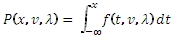# SQL Server non-central T inverse distribution function

NCTINV

Updated: 31 July 2010

Use NCTINV to calculate the inverse of the lower cumulative distribution function of the non-central T distribution.

The formula for the lower cumulative distribution function is:Syntax
SELECT [wctStatistics].[wct].[NCTINV] (
<@P, float,>
,<@DF, float,>
,<@Lambda, float,>)
Arguments
@CDF
is cumulative distribution function. @CDF is an expression of type float or of a type that implicitly converts to float
@DF
degrees of freedom. @DF is an expression of type float or of a type that implicitly converts to float
@Lambda
is the non-centrality parameter. @Lambda is an expression of type float or of a type that implicitly converts to float
Return Types
float
Remarks
·         @CDF must be greater than zero and less than one (0 < @CDF < 1).
·         @DF must be greater than zero (@DF > 0).
·         @Lambda must be greater than or equal to zero (@Lambda > 0).
Examples
Calculate the lower cumulative distribution function:
SELECT wct.NCTCDF(1,3,3)
This produces the following result
----------------------
0.0270004801469904

(1 row(s) affected)

Calculate the inverse:
SELECT wct.NCTINV(0.0270004801469904,3,3)
This produces the following result
----------------------
0.999999999999998

(1 row(s) affected)

### SupportCopyright 2008-2021 Westclintech LLC         Privacy Policy        Terms of Service